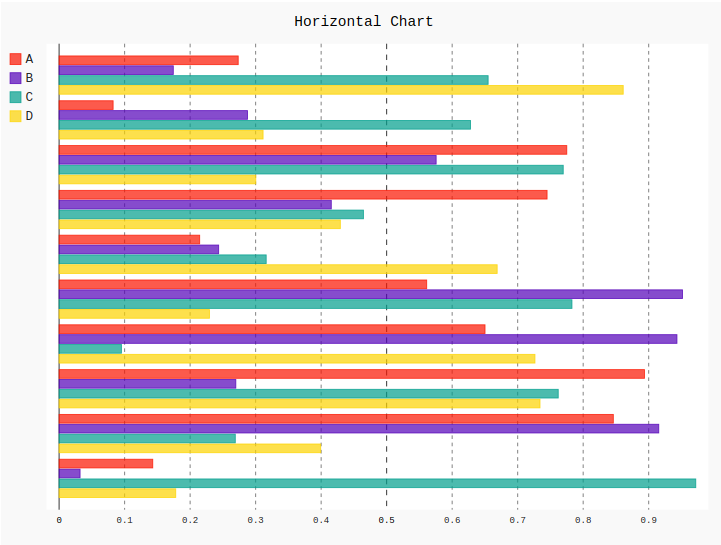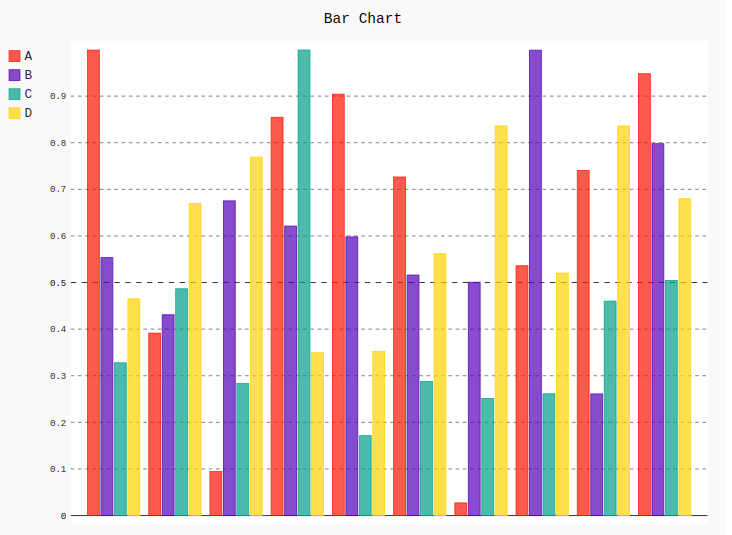# Bar Chart in Pygal

Pygal is a Python module that is mainly used to build SVG (Scalar Vector Graphics) graphs and charts. SVG is a vector-based graphics in the XML format that can be edited in any editor. Pygal can create graphs with minimal lines of code that can be easy to understand and write.

## Bar Chart

A bar chart or a graph is which present the data in the categorical form with rectangular bars with heights or lengths proportional to the values which represent in a graph. The bars can be plotted vertically or horizontally. A vertical bar chart is sometimes known as a column chart. One axis of the chart shows the specific categories being compared and the other axis represents a measured value.

• Horizontal bar graph is a graph which represents the data horizontally. All the data values in the horizontal graph are shown in the horizontal axis. This type of column graph helps to understand the data more effectively as the data is parallel to each other. It can be created using the HorizontalBar() method.
• Syntax:
`line_chart = pygal.HorizontalBar()`
• Example :

## Python3

 `# importing pygal` `import` `pygal` `import` `numpy`     `# creating the chart object` `horizontal_chart ``=` `pygal.HorizontalBar()`   `# naming the title` `horizontal_chart.title ``=` `'Horizontal Chart'`          `# Random data` `horizontal_chart.add(``'A'``, numpy.random.rand(``10``))` `horizontal_chart.add(``'B'``, numpy.random.rand(``10``))` `horizontal_chart.add(``'C'``, numpy.random.rand(``10``))` `horizontal_chart.add(``'D'``, numpy.random.rand(``10``))`   `horizontal_chart`

• Output:• Vertical bar graph displays the data by using vertical bars going up from the bottom, whose lengths are proportional to the quantities they represent. It can be used when one axis cannot have a numerical scale. A basic simple bar graph is very useful when presenting the series of data over time. It can be created using the Bar() method.
• Syntax:
`line_chart = pygal.Bar()`
• Example:

## Python3

 `# importing pygal` `import` `pygal` `import` `numpy`     `# creating the chart object` `bar_chart ``=` `pygal.Bar()`   `# naming the title` `bar_chart.title ``=` `'Bar Chart'`          `# Random data` `bar_chart.add(``'A'``, numpy.random.rand(``10``))` `bar_chart.add(``'B'``, numpy.random.rand(``10``))` `bar_chart.add(``'C'``, numpy.random.rand(``10``))` `bar_chart.add(``'D'``, numpy.random.rand(``10``))`   `bar_chart`

• Output:Whether you're preparing for your first job interview or aiming to upskill in this ever-evolving tech landscape, GeeksforGeeks Courses are your key to success. We provide top-quality content at affordable prices, all geared towards accelerating your growth in a time-bound manner. Join the millions we've already empowered, and we're here to do the same for you. Don't miss out - check it out now!